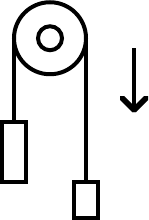427 pág.

# azdoc.tips-solutions-manual-fundamentalsofthermodynamicssonntag-borgnakke-vanwylen

DisciplinaTermodinâmica12.496 materiais232.465 seguidores
Pré-visualização427 páginas
2-1
CHAPTER 2
The correspondence between the problem set in this fifth edition versus the
problem set in the 4'th edition text. Problems that are new are marked new and
those that are only slightly altered are marked as modified (mod).
New Old New Old New Old
1 4 mod 21 13 4 1 E 33E mod
2 new 22 14 42 E 34E mod
3 new 23 15 4 3 E 3 5 E
4 7 mod 24 17 44E 36E
5 2 mod 25 18 45E 37E
6 new 26 new 4 6 E 3 8 E
7 new 27 19 4 7 E 3 9 E
8 new 28 20 4 8 E 4 0 E
9 5 mod 29 21 49E 41E
10 6 30 22
11 8 mod 31 23
12 new 32 24
13 9 mod 33 new
14 10 mod 34 25 mod
15 11 35 26 mod
16 new 36 27 mod
17 new 37 28
18 16 mod 38 29
19 new 3 9 E 31E mod
20 12 40E 32E
2-2
2.1 The “standard” acceleration (at sea level and 45° latitude) due to gravity is 9.80665
m/s2. What is the force needed to hold a mass of 2 kg at rest in this gravitational
field ? How much mass can a force of 1 N support ?
Solution:
ma = 0 = F = F - mg
F
= mg = 2 × 9.80665 = 19.613 N
F = mg => m = F/g = 1 / 9.80665 = 0.102 kg
2.2 A model car rolls down an incline with a slope so the gravitational “pull” in the
direction of motion is one third of the standard gravitational force (see Problem
2.1). If the car has a mass of 0.45 kg. Find the acceleration.
Solution:
ma = F = mg / 3
a = mg / 3m = g/3 = 9.80665 / 3 = 3.27 m/s2
2.3 A car drives at 60 km/h and is brought to a full stop with constant deceleration in 5
seconds. If the total car and driver mass is 1075 kg. Find the necessary force.
Solution:
Acceleration is the time rate of change of velocity.
ma = F ; a = dV / dt = (60 × 1000) / (3600 × 5) = 3.33 m/s2
Fnet = ma = 1075 × 3.333 = 3583 N
2.4 A washing machine has 2 kg of clothes spinning at a rate that generates an
acceleration of 24 m/s2. What is the force needed to hold the clothes?
Solution:
F = ma = 2 kg × 24 m/s2
= 48 N
2.5 A 1200-kg car moving at 20 km/h is accelerated at a constant rate of 4 m/s2 up to a
speed of 75 km/h. What are the force and total time required?
Solution:
a = dV / dt => t = dV/a = [ ( 75 20 ) / 4 ] × ( 1000 / 3600 )
t = 3.82 sec ; F = ma = 1200 × 4 = 4800 N2-3
2.6 A steel plate of 950 kg accelerates from rest with 3 m/s
2 for a period of 10s. What
force is needed and what is the final velocity?
Solution:
Constant acceleration can be integrated to get velocity.
a = dV / dt => dV = a dt => V = a t = 3 × 10 = 30 m/s
V = 30 m/s ; F = ma = 950 × 3 = 2850 N
2.7 A 15 kg steel container has 1.75 kilomoles of liquid propane inside. A force of 2
kN now accelerates this system. What is the acceleration?
Solution:
ma = F a = F / m
m = msteel + mpropane = 15 + (1.75 × 44.094) = 92.165 kg
a = 2000 / 92.165 = 21.7 m/s2
2.8 A rope hangs over a pulley with the two equally long ends down. On one end you
attach a mass of 5 kg and on the other end you attach 10 kg. Assuming standard
gravitation and no friction in the pulley what is the acceleration of the 10 kg mass
when released?
Solution:
Do the equation of motion for the mass m2 along the
downwards direction, in that case the mass m 1 moves
up (i.e. has -a for the acceleration)
m2 a = m2 g m
1 g m1a
(m1 + m2 ) a = (m2 m
1 )g
This is net force in motion direction
a = (10 5) g / (10 + 5) = g / 3 = 3.27 m/s2
g
1
2
2.9 A bucket of concrete of total mass 200 kg is raised by a crane with an acceleration
of 2 m/s2 relative to the ground at a location where the local gravitational
acceleration is 9.5 m/s2. Find the required force.
Solution:
F = ma = Fup mg
Fup = ma + mg = 200 ( 2 + 9.5 ) = 2300 N